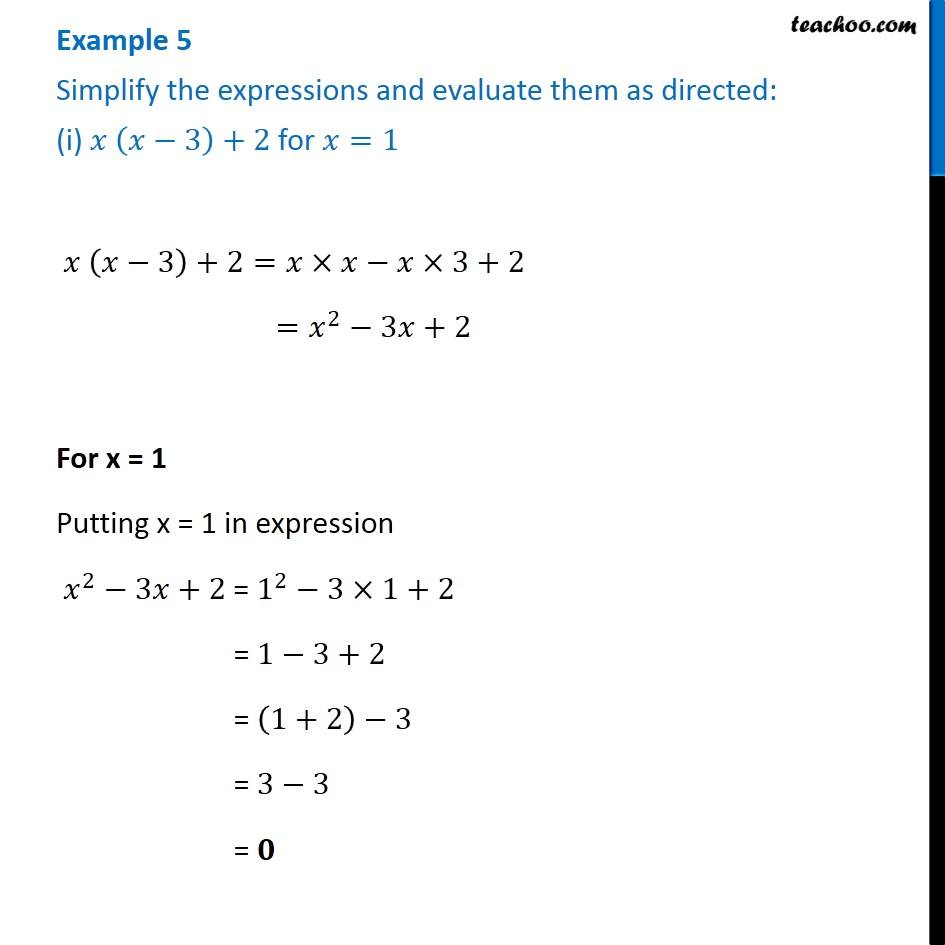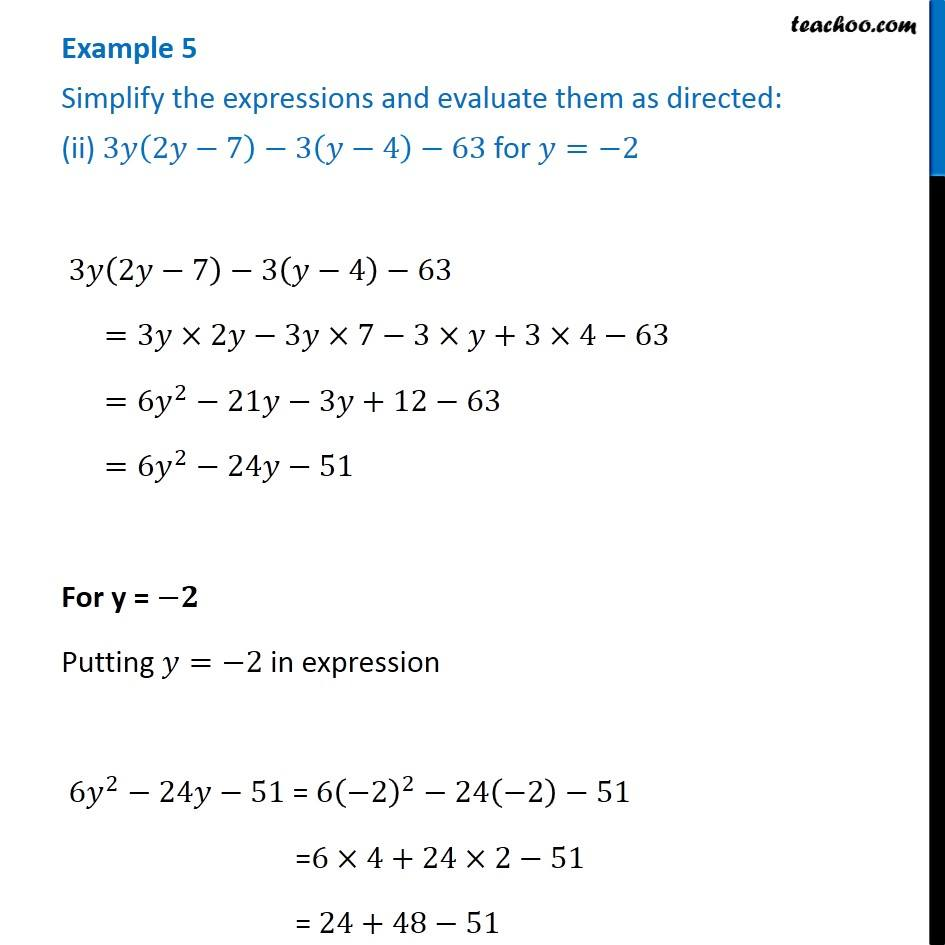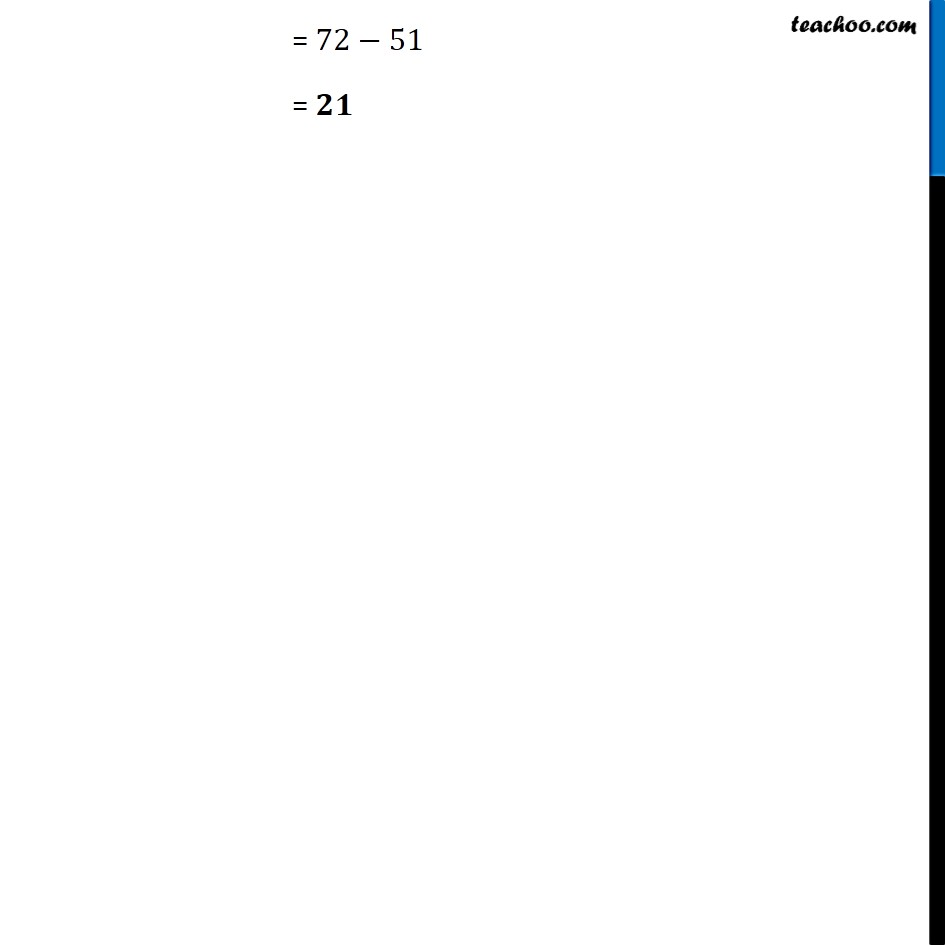Subscribe to our Youtube Channel - https://you.tube/teachoo

1. Chapter 9 Class 8 Algebraic Expressions and Identities
2. Serial order wise
3. Examples

Transcript

Example 5 Simplify the expressions and evaluate them as directed: (i) 𝑥 (𝑥−3)+2 for 𝑥=1 𝑥 (𝑥−3)+2=𝑥×𝑥−𝑥×3+2 =𝑥^2−3𝑥+2 For x = 1 Putting x = 1 in expression 𝑥^2−3𝑥+2 = 1^2−3×1+2 = 1−3+2 = (1+2)−3 = 3−3 = 𝟎 Example 5 Simplify the expressions and evaluate them as directed: (ii) 3𝑦(2𝑦−7)−3(𝑦−4)−63 for 𝑦=−2 3𝑦(2𝑦−7)−3(𝑦−4)−63 =3𝑦×2𝑦−3𝑦×7−3×𝑦+3×4−63 =6𝑦^2−21𝑦−3𝑦+12−63 =6𝑦^2−24𝑦−51 For y = −𝟐 Putting 𝑦=−2 in expression 6𝑦^2−24𝑦−51 = 6(−2)^2−24(−2)−51 =6×4+24×2−51 = 24+48−51 = 72−51 = 𝟐𝟏

Examples